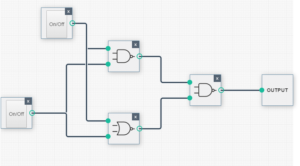# Digital Electronics VIVA QUESTIONS

The following are a few commonly asked viva questions for Digital Electronics experiments:

1. What is Boolean Algebra?
2. Define a logi-gate. Why is it called so?
3. What is positive logic and negative logic?
4. Define a truth table.
5. Define an analog signal and a digital signal.
6. List out the IC’s for various logic operations like NAND, NOR, XOR, OR, AND etc.
7. What is De Morgan’s theorem?
8. What is the use of De Morgan’s theorem?
9. Implement the various logics using the NOR gate.
10. Implement the various logic gates using the NAND gate.
11. Give the difference between a Full and Half- Adder.
12. Write down the Truth table for the following logic circuit.  Also name the logic gate obtained.It is an OR gate

Check out this useful tool:

13. What is the value of Y=A.B+A if
(i) A=B=1
(ii)A=0, B=1
(iii)A=1, B=0.
14. What is the difference between half and full-subtractor?

You might wanna check the following tools out:
https://scratch.mit.edu/projects/785418/
http://www.digikey.com/schemeit/project/

https://www.bragitoff.com/2014/10/top-7-books-studying-digital-systems-design-bachelor-courses/

https://www.bragitoff.com/2015/01/ttl-ics-74xx-series-appendix-pin-diagrams/[wpedon id="7041" align="center"]